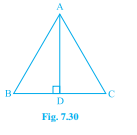"
">

# In $\triangle \mathrm{ABC}, \mathrm{AD}$ is the perpendicular bisector of $\mathrm{BC}$ (see Fig. 7.30). Show that $\triangle \mathrm{ABC}$ is an isosceles triangle in which $\mathrm{AB}=\mathrm{AC}$."

Given:

In $\triangle ABC, AD$ is the perpendicular bisector of $BC$.

To do:

We have to show that $\triangle ABC$ is an isosceles triangle in which  $AB=AC$.

Solution:

Let us consider $\triangle ADB$ and$\triangle ADC$,

We know that,

According to Rule of Side-Angle-Side Congruence:

Triangles are said to be congruent if any pair of corresponding sides and their included angles are equal in both triangles.

Since $AD$ is the common side of both the triangles,

We get,

$AD=DA$

This implies,

$\angle ADB= \angle ADC$

Since $AD$ is  a perpendicular bisector of $\triangle ABC$ we get,

$BD=CD$

Therefore,

$\triangle ADB \cong \triangle ADC$

We also know

From corresponding parts of congruent triangles: If two triangles are congruent, all of their corresponding  sides must be equal.

Therefore,

$AB=AC$.

Updated on: 10-Oct-2022

31 Views